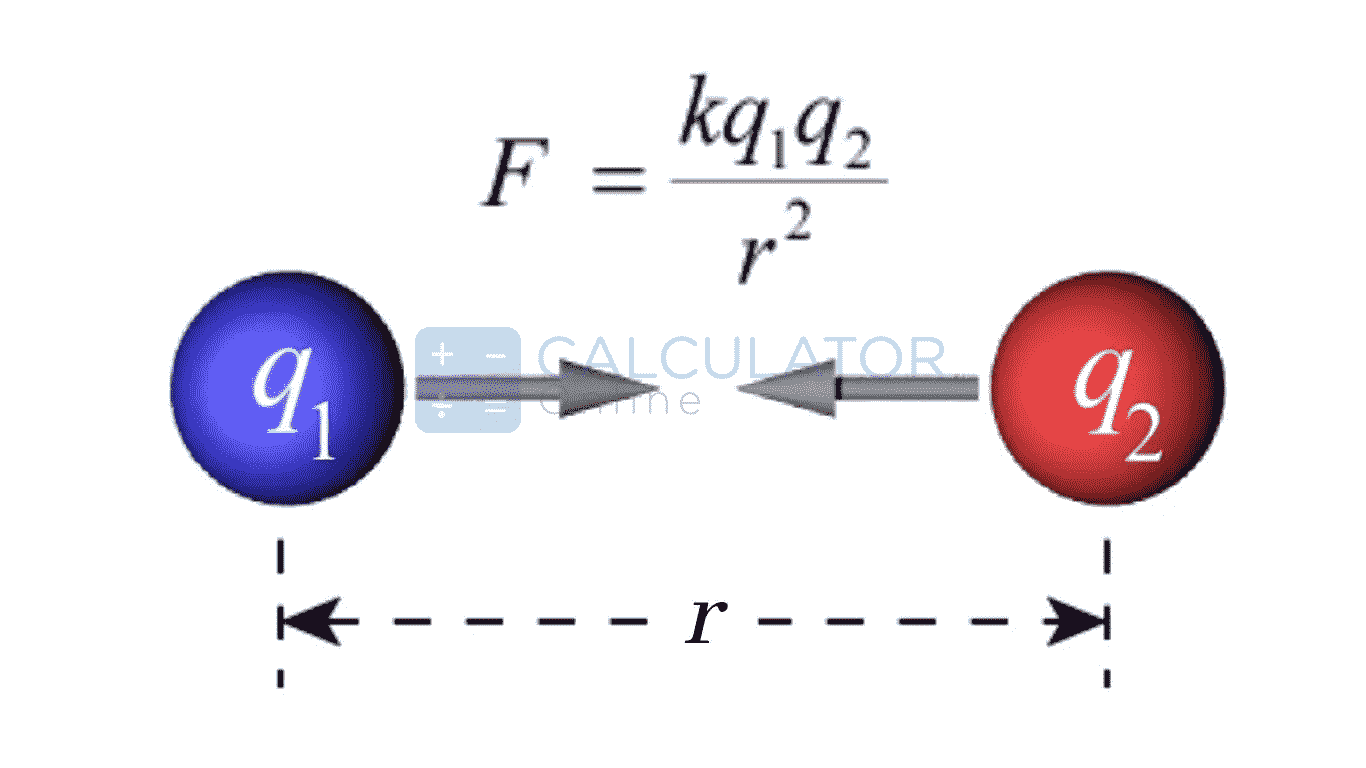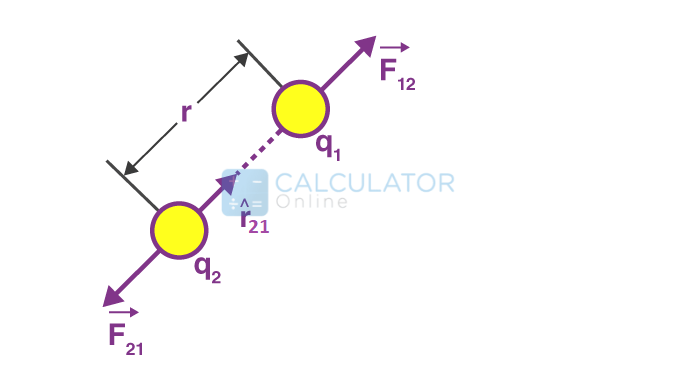•Sign In
• Hire UsUh Oh! It seems you’re using an Ad blocker!

We always struggled to serve you with the best online calculations, thus, there's a humble request to either disable the AD blocker or go with premium plans to use the AD-Free version for calculators.

Or# Coulomb's Law Calculator

Charges are:

I want to calculate

I want to calculate

Charge (q₁ & q₂)

Charge 1 (q₁)

Charge 2 (q₂)

Distance (r)

Force (F)

Coulomb's Constant (Ke)

x109 N⋅m²/C²

Table of Content

 1 What is priceeight Class? 2 priceeight Class Chart: 3 How to Calculate priceeight Density (Step by Step): 4 Factors that Determine priceeight Classification: 5 What is the purpose of priceeight Class? 6 Are mentioned priceeight Classes verified by the officials? 7 Are priceeight Classes of UPS and FedEx same?

Get the Widget!

Add this calculator to your site and lets users to perform easy calculations.

Feedback

How easy was it to use our calculator? Did you face any problem, tell us!

The online free Coulomb’s Law Calculator provides the step by step solution to calculate the force of attraction or repulsion between two charges, their electric charge, according to the distance between the charges.

## The Coulomb Law:

The Coulomb Law states that the force of attraction or repulsion is directly proportional to the product of the charges and inversely proportional to Square of the distance between them”

The Coulomb Law calculates the electrostatic force acting between two charges. The force is always acting along the straight line that join the two charges (q₁q₂) and the force act along the square of the shortest distance (r²) between the charges:The Coulomb’s law Formula is as follows:

F = ke[q₁q₂/r²]

Where

F=The Electrostatic Force  between the charges in Newtons(N)

q₁= It is the magnitude of the first charge in Coulombs(C)

q₂= It is the magnitude of the second charge in Coulombs(C)

r= The shortest distance between the charges in Meters(m)

ke= It the Coulomb’s constant and its value is equal to 8.98755 × 10⁹ N·m²/C².

The values of the “ke” have been inserted into the Coulomb’s law calculator, and you have no need to remember the accurate value of the “ke”. The electrostatic force calculator automatically finds the value of the coulomb’s constant value.

### The unit of electric charge:

The SI unit of the electric charge is Coulombs(denoted by C).

1 Coulomb is a charge that is transported by the constant current of 1 Ampere during 1 Second”.

How to calculate coulombs?

1 Coulomb =1 Ampere×1 Second

Where Ampere is the unit of the current and we can measure the current or  Ampere by the Ohm’s calculator.

### Historical perspective of the Coulomb’s Law:

Coulomb was a French physicist and his name was Charles Augustin de Coulomb. He presented the Coulomb’s law formula in 1785 to define the force of attractions or repulsion between two electrical charges.He presented an equation of the force showing the force of attraction or repulsion between two bodies and it is known as the Coulomb’s electrostatic force formula

### Coulomb’s law in vector form:

We can present Coulomb’s law in vector form to define  force between two charges as both the charges are exerting  opposite and equal forces on each other. We need to find the projections of the vectors and their force of attraction or repulsion of each other. For this we use the vector projection calculator to find the projection of the vectors.Now consider two charges q1 and q2 , these charges are exerting a force of  F12  and q2 is exerting a force of F21  on the charge q1

We can represent both the forces by:

$$\begin{array}{l}\left( ie\,\,\,{{{\vec{F}}}_{12}}=-{{{\vec{F}}}_{21}} \right)\end{array}$$

The electrostatic force formula for two charges is as follows:

$$\begin{array}{l}{{\vec{F}}_{12}}=\frac{1}{4\pi {{\in }_{0}}}\frac{{{q}_{1}}{{q}_{2}}}{r_{12}^{2}}{{\hat{r}}_{12}}; \;\;{{\vec{F}}_{12}}=-{{\vec{F}}_{21}}\end{array}$$

The resultant force due to number of point charges can be represented as:

$$\begin{array}{l}\vec{F}={{\vec{F}}_{1}}+{{\vec{F}}_{2}}+{{\vec{F}}_{3}}+……\end{array}$$

By implementing this above calculation,it is possible to implement electrostatic force formula on the number of point charges.

### The limitations of the Coulomb’s Law:

There are certain limitations of the  Coulomb’s Law:

• The Coulomb’s Law  is applicable only on point charges at rest.
• We can’t implement the electrostatic force formula on the charges when they are moving as we can’t find the distance between them.
• The coulomb’s law formula would not able to provide the perfect result when we are dealing with huge charged objects

### Working of the Coulomb’s Law Calculator:

The Force of attraction or repulsion  between two charges can be measured by the Coulombs law calculator:

Input:

• Enter the charges.
• Enter the distance between the charges
• Hit the calculate button to find force.

Output:

The coulomb’s law calculator do the following calculations:

• It find the force of attraction or repulsion between to charges
• Display the step by step calculations.

### Why do charges repel each other?

Like charges repel each other as their forces are directed against each other. This is the main reason the like charges produce repellent force to each other.The electrical force calculator finds the force of repulsion between the like charges.

### What is the SI unit of electrostatic force?

The electrostatic force is measured in Newton(N).The other units of  electrostatic force are (Kilo Newton) kN,(Milli Newton) mN, (Mega Newton) MN, (pounds-force) lbf, Giga Newton) GN, (Tera Newton) TN,(poundals) pdl

### What is meant by esu and emu?

The “esu” denotes the “electrostatic unit” and it is also represented as prefix “stat”and read as statcoulomb. The emu denotes electromagnetic unit and is also given the prefix “ab” known as abcoulomb.

### What is a common example of the  electrostatic force?

The common example of the electrostatic force is when you run a comb in our dry hairs and attract the pieces of papers by it.

### What does “Q” represent in the electric field?

We place the charge “Q” to check the sphere of the electric field and it is called the test charge in the electric field. We can find the strength of the electric field by the coulomb force calculator.We can find the electric field by the electric field calculator.

### Conclusion:

The coulomb’s law formula has great application in modern day life from the Xerox machines to the Laser printer, it is also utilized for the powder coating. In these machines charged particles are used in the printing process. The coulomb’s law calculator is used to find the force of attraction or repulsion between two objects in installing them in various machines.

### References:

From the source of Wikipedia: Coulomb, Definition

From the source of Britannica:Charles-Augustin de Coulomb,Coulomb force